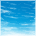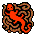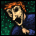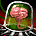## cw v6.2 math precision

cancel
Showing results for
Did you mean:

## cw v6.2 math precision

3,623 ViewsContributor I

Hello,

I'm working with cw 6.2, and trying do make some trigonometric calculations. The target is a MCF51qe.

For exemple, when I do this:

sin((double)0.86217507327430);

I obtain 0.75926020631887015.

In matlab, the same sinus gives 0.75925986842193.

The difference is not so big, but I have difference for each sin/cos calculation, and the result is used to found the distance between 2 GPS points. The distance is given using an acos function but the argument of the acos is >1, due to precision problems; so it can't be calculated. I'm sure of the formulas I'm using, as I checked with a computer and the problem doesn't appear (argument of acos is always<1).

In cw, I have included this libs:

fp_coldfire_nodiv.a

C_4i_CF_RegABI_MATH_MSL.a

C_4i_CF_RegABI_Runtime.a

I've tried other libs but without success.

Someone has an idea?

Labels (1)
• ### General

Tags (1)
32 Replies
241 ViewsContributor III

I have CW10.1 loaded,    and having many problems with Windows 7 64 bit system

one aspect is that I can define a project  S08JM60 for example and set up the full CPU - - all timers etc.

all is fine then close down for the night and come back and find that the CPU does not show any  more - - - -

any ideas about what causes this.

also hint for people using external clock with CW 10.1.  first I have to setup the external clock pin in the G port

(change port from 8 bits to individual pins)  then can set the CPU clock module to select external pin and only then does the option for internal clock provide the ability to select external clock - - - - - go figure.

Jim P

241 ViewsNXP Employee

Are you using Processor Expert? The settings are stored in the *.pe file. Could it be that this file has not been saved? You can force saving the file with CTRL-S (with say the component inspector open/selected) or with Project > Close menu.

BK

241 ViewsContributor III

I typically close by hitting the upper right exit box [X]  and CW asks to save the files.

I have found many problems with PE so simply use device init.

Also I have found in the past the PE really does nothing for me. And adds more lines of code to be processed

instead of simply writting to the I/O port as    Port |= 0x40;

and I write macro's for this    #define for the function of each - as  #define Motor1_On  Port |= 0x40.

makes code simple and still very fast.

with PE, I do not get the CPU showing - - with Device init, I get CPU showing but loose it the next time I load the project.

Will try saving with project close next time and see what happens.  Would make my life a lot easier.

Jim P

241 ViewsContributor III

my experince with FP is that single precision is about 7 to 8 digits max resolution/accuracy depending

Sin does quite a bit of math so would expect 6 digits to be the max resolution / accuracy

what causes the problem is that the numbers are displayed to way to many digits - - - so makes it look like errors are present.

double precision give in the area of 15 digits of resolution/accuracy

one way to verify this - - simply add to the sin result 1e-10 and see if the result changes - - - - bet you that it will not change as a single floating point number does not have the resolution (number of bits to hold this small of a value)

Jim P

241 ViewsContributor III

I don't know about your compiler library (I use 9s12 ) but the same people most likely did yours as did mine,

#if !_SIN_HUGE

...

#else

#ifdef EXTENDED_PRECISION_COSSIN

#endif

... //more precision than original here

#endif

add this to your compiler options or define it in you first file and maybe the precision will come in.

#define _SIN_HUGE

#define EXTENDED_PRECISION_COSSIN

note you may get enough accuracy from _SIN_HUGE alone and you may not need the EXTENDED_PRECISION_COSSIN.

To me the "_" in "_SIN_HUGE" means that it is a system generated definition so you may have to check you options instead of forcing it like I did above.

Check your installation and look at the source file for the math.c file and dig in it to see if you have a conditional compile as well.  Then be sure to do a clean compile by removing all of the object files first.

... (my path for example) ...

C:\PROGRA~1\FREESC~1\CWFORH~1.5\lib\hc12c\src

note math.c is for double

mathf.c is for float

241 ViewsContributor I

Thanks for your cosinus finction Emac, it will be usefull.

I'm quite lost with the libraries, as I'm not used to work with this; but I'm still looking if there is something about precision.

241 ViewsContributor I

Hi Sanahuja,

I am also facing the similar problem which u were facing, i want to find the distance between 2 GPS points.

I have used the code of sin, cos functions(double precision),which Emac had posted & its working. But i also need the acos function(double precision). So please post the code acos function.

241 ViewsContributor III

I'll see what I can do about an acos function.241 ViewsContributor III
`/*This formula uses taylor integration expansion and the identity function to evaluatethe inverse cosine over the range of -1 to 1 (and improve errors by angle addition.)Error should be less than ~ 5.1e -9Maclaurin series expansion using f(c) where c = 0.f(x) = f(c)+f'(c)x + f''(c)x/2! + f'''(c)x/3! ....constantssqrt(3) 1.7320508075688800000E+00 pi/3 1.0471975511966000000E+00pi 3.1415926535897900000E+00 pi/2 1.5707963267949000000E+00constant polynomial termsa0 1.6666666666666700000E-01  =    1/(3*2)a1 4.5000000000000000000E-01  =  3^2/(4*5)a2 5.9523809523809500000E-01  =  5^2/(7*6)a3 6.8055555555555600000E-01  =  7^2/(9*8)a4 7.3636363636363600000E-01  =  9^2/(11*10)a5 7.7564102564102600000E-01  = 11^2/(13*12)a6 8.0476190476190500000E-01  = 13^2/(15*14)a7 8.2720588235294100000E-01  = 15^2/(17*16)a8 8.4502923976608200000E-01  = 17^2/(19*18)Used angle addition to reduce errors as x approaches -1 and +1Algorithem cases.if x>1  error .if x= 1 f(1) = 0 .if 1>x>.5 x' = .5*(  -1*(3^.5)*(1 -x)^.5   + x )   , f(x)=f(x')-pi/3 .if .5 >= x >=-.5 x=f(x) .if -1<x<-.5 x' = .5*(  (3^.5)*(1 -x)^.5   + x )   , f(x)=f(x')+pi/3 .if x=-1 f(-1) = pi .if x<-1 error f(x) = pi/2 -x(1+a0*x^2*(1+a1*x^2*(1+a2*x^2*(1+a3*x^2*(1+a4*x^2*(1+a5*x^2*(1+a6*x^2*(1+a7*x^2*(1+a8*x^2))))))))))    Error is less than 5.1e -9  f(x) = pi/2 -x(1+a0*x^2*(1+a1*x^2*(1+a2*x^2*(1+a3*x^2*(1+a4*x^2*(1+a5*x^2*(1+a6*x^2*(1+a7*x^2*(1+a8*x^2))))))))))  I found excellent site for other functions at http://mathworld.wolfram.com/MaclaurinSeries.htmlafter working hard on this function independeantly.*/#include <math.h>double myacos(double x);double myacos(double x){  //constants  const double pi              = 3.14159265358979000E+00;  const double pi_over_2       = 1.57079632679490000E+00;  const double pi_over_3       = 1.0471975511966000000E+00;  const double squareRootThree = 1.7320508075688800000E+00;                   const double acosPolynomialTerms=  {    1.6666666666666700000E-01,  //=    1/(3*2)    4.5000000000000000000E-01,  //=  3^2/(4*5)    5.9523809523809500000E-01,  //=  5^2/(7*6)      6.8055555555555600000E-01,  //=  7^2/(9*8)      7.3636363636363600000E-01,  //=  9^2/(11*10)      7.7564102564102600000E-01,  //= 11^2/(13*12)      8.0476190476190500000E-01,  //= 13^2/(15*14)      8.2720588235294100000E-01,  //= 15^2/(17*16)      8.4502923976608200000E-01   //= 17^2/(19*18)  };  double result;  double finalAddition;  double innerTerm;#define MY_DOUBLE_ERROR_IS_INFINITY 3.402823466E+38  //.if x>1  error   //.if x<-1 error   //how do you want to handle an error (throw exception?)  if(x > 1.0)  {    return(MY_DOUBLE_ERROR_IS_INFINITY);  }  if(x < -1.0)  {    return(MY_DOUBLE_ERROR_IS_INFINITY);  }  //.if x== 1 f(1) = 0   //.if x== -1 f(-1) = pi  if(x == 1.0)  {    return(0.0);  }  if(x == -1.0)  {    return(pi);  }  finalAddition = pi_over_2;  innerTerm = x*x;    //used angle addition to rotate the x,y coordinates by pi/3 to improve error  //.if 1>x>.5 x' = .5*(  -1*(3^.5)*(1 -x)^.5   + x )   , f(x)=f(x')-pi/3   //.if -1<x<-.5 x' = .5*(  (3^.5)*(1 -x)^.5   + x )   , f(x)=f(x')+pi/3   if(( x < 1.0  ) && (  x > 0.5 )   )  {    x -=  ( sqrt(x) * squareRootThree) ;    x = x * 0.5;    innerTerm = x*x;    finalAddition -= pi_over_3;  }else if(( x > -1.0  ) && (  x < -0.5 )   )  {    x +=  ( sqrt(x) * squareRootThree) ;    x = x * 0.5;    innerTerm = x*x;    finalAddition += pi_over_3;  }  //f(x) = pi/2 -x(1+a0*x^2*(1+a1*x^2*(1+a2*x^2*(1+a3*x^2*  //           (1+a4*x^2*(1+a5*x^2*(1+a6*x^2*(1+a7*x^2*(1+a8*x^2))))))))))    result = acosPolynomialTerms*innerTerm        + 1.0;  result = acosPolynomialTerms*innerTerm*result + 1.0;  result = acosPolynomialTerms*innerTerm*result + 1.0;  result = acosPolynomialTerms*innerTerm*result + 1.0;  result = acosPolynomialTerms*innerTerm*result + 1.0;  result = acosPolynomialTerms*innerTerm*result + 1.0;  result = acosPolynomialTerms*innerTerm*result + 1.0;  result = acosPolynomialTerms*innerTerm*result + 1.0;  result = acosPolynomialTerms*innerTerm*result + 1.0;  result = -1.0*x*result;  result += finalAddition;  return(result);}`

241 ViewsContributor I

Hi Emac ,

How r u ?

Hope u r fine...

I have used the trigonometric functions which u had posted. All functions are working fine. But, i am facing problem with sqrt function, sqrt function which i am using supports floating point value because of which i am getting some difference in values.

Could you please share me the sqrt function which supports double precision floating point value.

241 ViewsContributor III

Hi Shiva,

I am doing fine.

Thanks for the feedback from above.

I don't know what your particular issue with the sqrt function is.  You claim the other functions are working but I know the acos function uses the sqrt as well.

In any case I ported the sqrt function from the Sun Microsystems original

http://www.netlib.org/fdlibm/index.html site, so it is not my creation but is free to use under the guidelines spelled forth in the header.

`               /* @(#)e_sqrt.c 1.3 95/01/18 *//* * ==================================================== * Copyright (C) 1993 by Sun Microsystems, Inc. All rights reserved. * * Developed at SunSoft, a Sun Microsystems, Inc. business. * Permission to use, copy, modify, and distribute this * software is freely granted, provided that this notice  * is preserved. * ==================================================== *//* __ieee754_sqrt(x) * Return correctly rounded sqrt. *           ------------------------------------------ *      |  Use the hardware sqrt if you have one | *           ------------------------------------------ * Method:  *   Bit by bit method using integer arithmetic. (Slow, but portable)  *   1. Normalization * Scale x to y in [1,4) with even powers of 2:  * find an integer k such that  1 <= (y=x*2^(2k)) < 4, then *  sqrt(x) = 2^k * sqrt(y) *   2. Bit by bit computation * Let q  = sqrt(y) truncated to i bit after binary point (q = 1), *      i        0 *                                     i+1         2 *     s  = 2*q , and y  =  2   * ( y - q  ).  (1) *      i      i            i                 i *                                                         * To compute q    from q , one checks whether  *      i+1       i                        * *         -(i+1) 2 *   (q + 2      ) <= y.   (2) *          i *             -(i+1) * If (2) is false, then q   = q ; otherwise q   = q  + 2      . *           i+1   i             i+1   i * * With some algebric manipulation, it is not difficult to see * that (2) is equivalent to  *                             -(i+1) *   s  +  2       <= y   (3) *    i                i * * The advantage of (3) is that s  and y  can be computed by  *          i      i * the following recurrence formula: *     if (3) is false * *     s     =  s  , y    = y   ;   (4) *      i+1      i   i+1    i * *     otherwise, *                         -i                     -(i+1) *     s   =  s  + 2  ,  y    = y  -  s  - 2    (5) *           i+1      i          i+1    i     i *     * One may easily use induction to prove (4) and (5).  * Note. Since the left hand side of (3) contain only i+2 bits, *       it does not necessary to do a full (53-bit) comparison  *       in (3). *   3. Final rounding * After generating the 53 bits result, we compute one more bit. * Together with the remainder, we can decide whether the * result is exact, bigger than 1/2ulp, or less than 1/2ulp * (it will never equal to 1/2ulp). * The rounding mode can be detected by checking whether * huge + tiny is equal to huge, and whether huge - tiny is * equal to huge for some floating point number "huge" and "tiny". *   * Special cases: * sqrt(+-0) = +-0  ... exact * sqrt(inf) = inf * sqrt(-ve) = NaN  ... with invalid signal * sqrt(NaN) = NaN  ... with invalid signal for signaling NaN * * Other methods : see the appended file at the end of the program below. *--------------- */#ifndef MYSQRT_HEADER#define MYSQRT_HEADER#include <math.h>#ifdef __STDC__static const double one = 1.0, tiny=1.0e-300;#elsestatic double one = 1.0, tiny=1.0e-300;#endifdouble ieee754_sqrt(double x){ double z; signed long int  sign            = 0x80000000;  unsigned long int r,t1,s1,ix1,q1; signed long int   ix0,s0,q,m,t,i;  signed long       myLong;union DATATYPE_DOUBLE_INT// Declare union type{   double      doubleValue;   long int    intValue;};// Optional declaration of union variable    union DATATYPE_DOUBLE_INT tempValue;  tempValue.doubleValue = x;  myLong = x;    //ix0 = __HI(x);   /* high word of x */ //ix1 = __LO(x);  /* low word of x */  //big endian  ix0 = tempValue.intValue;   //HI  ix1 = tempValue.intValue;   //LO  //little endian  //ix0 = tempValue.intValue; //HI  //ix1 = tempValue.intValue; //LO    /* take care of Inf and NaN */ if((ix0 & 0x7ff00000) == 0x7ff00000)  {         ix0 =                   0x7ff00000;        ix1 =                   0;        //little endian        //tempValue.intValue = ix0;  //HI        //tempValue.intValue = ix1;  //LO                //big endian        tempValue.intValue = ix0;  //HI        tempValue.intValue = ix1;  //LO        return tempValue.doubleValue;  /* sqrt(NaN)=NaN, sqrt(+inf)=+inf        sqrt(-inf)=sNaN */ }     /* take care of zero */ if(ix0<=0) {     if(((ix0 & (~sign)) | ix1) == 0)         return x;/* sqrt(+-0) = +-0 */     else if(ix0<0)      {      ix0 =                   0x7ff00000;        ix1 =                   0;        //little endian        //tempValue.intValue = ix0;  //HI        //tempValue.intValue = ix1;  //LO                //big endian        tempValue.intValue = ix0;  //HI        tempValue.intValue = ix1;  //LO        return tempValue.doubleValue;      }/* sqrt(-ve) = sNaN */ }    /* normalize x */ m = (ix0 >> 20); if(m == 0) {    /* subnormal x */     while(ix0 == 0)      {      m -= 21;      ix0 |= (ix1 >> 11); ix1 <<= 21;     }     for(i = 0;(ix0 & 0x00100000) == 0; i++) ix0 <<= 1;     m -= i - 1;     ix0 |= (ix1 >> (32 - i));     ix1 <<= i; } m -= 1023; /* unbias exponent */ ix0 = (ix0 & 0x000fffff) | 0x00100000; if(m&1){ /* odd m, double x to make it even */     ix0 += ix0 + ((ix1 & sign) >> 31);     ix1 += ix1; } m >>= 1; /* m = [m/2] */    /* generate sqrt(x) bit by bit */ ix0 += ix0 + ((ix1 & sign) >> 31); ix1 += ix1; q = q1 = s0 = s1 = 0; /* [q,q1] = sqrt(x) */ r = 0x00200000;  /* r = moving bit from right to left */ while(r != 0) {     t = s0 + r;      if(t <= ix0) {   s0   = t + r;   ix0 -= t;   q   += r;      }      ix0 += ix0 + ((ix1 & sign) >> 31);     ix1 += ix1;     r>>=1; } r = sign; while(r!=0) {     t1 = s1 + r;      t  = s0;     if((t < ix0) || ((t == ix0) && (t1 <= ix1)))      {       s1  = t1 + r;      if(((t1 & sign) == sign)&&(s1 & sign) ==0) s0 += 1;      ix0 -= t;      if (ix1 < t1) ix0 -= 1;      ix1 -= t1;      q1  += r;     }     ix0 += ix0 + ((ix1 & sign) >> 31);     ix1 += ix1;     r>>=1; }    /* use floating add to find out rounding direction */ if((ix0|ix1)!=0) {     z = one - tiny; /* trigger inexact flag */     if (z >= one)      {         z = one + tiny;         if (q1 == (unsigned)0xffffffff) { q1=0; q += 1;}        else if (z>one)         {            if (q1==(unsigned)0xfffffffe) q+=1;            q1+=2;         } else             q1 += (q1&1);     } } ix0 = (q>>1)+0x3fe00000; ix1 =  q1>>1; if ((q & 1) == 1) ix1 |= sign; ix0 += (m << 20);// __HI(z) = ix0;// __LO(z) = ix1;  //little endian//  tempValue.intValue = ix0;  //HI//  tempValue.intValue = ix1;  //LO  //big endian  tempValue.intValue = ix0;  //HI  tempValue.intValue = ix1;  //LO  z = (double) tempValue.doubleValue; return z;}#endif //MYSQRT_HEADER`

`    double ieee754_sqrt(double x);`

I compiled and tested it on my machine but quickly found that it matters if your system is big endian or little endian (MicroSoft studio.net, for example, ran little endian)

241 ViewsContributor III

Note on acos function above,

you will need to remove the declaration statement:

double myacos(double x);

You should put that statement in a seperate header file.

Otherwise, it will compile but give you goofy answers.

`I found excellent site for other functions at http://mathworld.wolfram.com/MaclaurinSeries.htmlafter working hard on this function independeantly.*/#include <math.h>double myacos(double x);`

You should then have a headr file called myacos.h

`//this is my header file myacos.hdouble myacos(double x);`

and the above snippet of code should now read:

`I found excellent site for other functions at http://mathworld.wolfram.com/MaclaurinSeries.htmlafter working hard on this function independeantly.*/#include <math.h>#include "myacos.h"`

maybe that is what was going wrong?

241 ViewsContributor I

Hi Emac,

Ya i have already taken care about it, but still not getting accurate values.

I guess  the sqrt function which i am using in acos function, supports the floating point  values, hence i think because of this, there might be the reason for getting non accurate values. So i will use the sqrt function which you had posted & will test...

241 ViewsContributor I

Hi Emac,

You are great.Thank U...

I used this acos function & checked, Its working fine.

Sorry for the delayed response. Thank U once again.

241 ViewsSpecialist I
241 ViewsContributor I

Hi kef,

Thank u for the response.

241 ViewsContributor III

In case you need a cos algorithem in the mean time.

`/*This formula uses taylor integration expansion and the identity function to evaluatecosine over the range of pi/2 to 3pi/2 (and by trig identities, -2pi to 2 pi)error should be less than ~ 1e-10Inspiration from pg 81 tailor series expansion from Numerical Methods fo Engineersby Chapra & Canale Mcgraw Hill publishersa = pi (in order to use trig identities)*/double mycos(double x){  const double multiplierMatrix[] ={-5.49450549450549450E-03, //  -1/(14*13)-7.57575757575757576E-03, //  -1/(12*11)-1.11111111111111111E-02, //  -1/(10*9)-1.78571428571428571E-02, //  -1/(8*7)-3.33333333333333333E-02, //  -1/(6*5)-8.33333333333333333E-02, //  -1/(4*3)-5.00000000000000000E-01 //  -1/(2*1)};  double result;  double finalSign;  double innerTerm;  const double pi_over_2       = 1.57079632679490000E+00;  const double pi              = 3.14159265358979000E+00;  const double three_pi_over_2 = 4.71238898038469000E+00;  const double twoPi           = 6.28318530717959000E+00;#define MY_DOUBLE_ERROR_IS_INFINITY 0x7ff0000000000000  //handle negative numbers  if(x < 0.0)  {    x= x + twoPi;  }  //how do you want to handle an error (throw exception?)  if(x >= twoPi)  {    return(MY_DOUBLE_ERROR_IS_INFINITY);  }  if(x < 0.0)  {    return(MY_DOUBLE_ERROR_IS_INFINITY);  }  if( x < pi_over_2  )  {    innerTerm = x*x;    finalSign = (double) +1.0;  }else if( x < three_pi_over_2 )  {    innerTerm = x - pi;    innerTerm = innerTerm*innerTerm;    finalSign = (double) -1.0;  }else  {    innerTerm = x - twoPi;    innerTerm = innerTerm*innerTerm;    finalSign = (double) +1.0;  }  result = multiplierMatrix*innerTerm        + 1.0;  result = multiplierMatrix*innerTerm*result + 1.0;  result = multiplierMatrix*innerTerm*result + 1.0;  result = multiplierMatrix*innerTerm*result + 1.0;  result = multiplierMatrix*innerTerm*result + 1.0;  result = multiplierMatrix*innerTerm*result + 1.0;  result = multiplierMatrix*innerTerm*result + 1.0;  result = result * finalSign;  return(result);}`

241 ViewsContributor I

Hi Emac,

Thanks for posting the codes of sin, cos functions(double precision). Could u please post the code of acos function(double precision).

241 ViewsContributor III

Final code

`/*This formula uses taylor integration expansion and the identity function to evaluatesine over the range of 0 to pi (and by trig identities, -2pi to 2 pi)error should be less than ~ 5e-10*/double mysin(double x){  double identityMatrix[]={-1.00000000000000000E+00,+1.00000000000000000E+00,-1.00000000000000000E+00,+1.00000000000000000E+00,-1.00000000000000000E+00,+1.00000000000000000E+00,-1.00000000000000000E+00};  double multiplierMatrix[] ={5.49450549450549450E-03, //  1/(14*13)7.57575757575757575E-03, //  1/(12*11)1.11111111111111111E-02, //  1/(10*9)1.78571428571428571E-02, //  1/(8*7)3.33333333333333333E-02, //  1/(6*5)8.33333333333333333E-02, //  1/(4*3)5.00000000000000000E-01  //  1/(2*1)};  double result;  double finalSign;  double innerTerm;  const double pi_over_2 = 1.57079632679490000E+00;  const double pi        = 3.14159265358979000E+00;  const double twoPi     = 6.28318530717959000E+00;#define MY_DOUBLE_ERROR_IS_INFINITY 0x7ff0000000000000  //handle negative numbers  if(x < 0.0)  {    x= x + twoPi;  }  //how do you want to handle an error (throw exception?)  if(x >= twoPi)  {    return(MY_DOUBLE_ERROR_IS_INFINITY);  }  if(x < 0.0)  {    return(MY_DOUBLE_ERROR_IS_INFINITY);  }  if( x < pi)  {    innerTerm = x -  pi_over_2;    innerTerm = innerTerm*innerTerm;    finalSign = (double) -1.0;  }else  {    innerTerm = x - pi -  pi_over_2;    innerTerm = innerTerm*innerTerm;    finalSign = (double) +1.0;  }  result = multiplierMatrix*innerTerm + identityMatrix;  result = multiplierMatrix*innerTerm*result + identityMatrix;  result = multiplierMatrix*innerTerm*result + identityMatrix;  result = multiplierMatrix*innerTerm*result + identityMatrix;  result = multiplierMatrix*innerTerm*result + identityMatrix;  result = multiplierMatrix*innerTerm*result + identityMatrix;  result = multiplierMatrix*innerTerm*result + identityMatrix;  result = result * finalSign;  return(result);}`

I don't know if moderator could link to this code instead of broken one from before241 ViewsContributor I

Thanks again Emac.

Your function is working well; I will search for the other trigonometric functions(cos, tan,acos,asin,atan), as I have the same problem with these functions.

I don't had the time to check the speed, but I will do it and let you know.